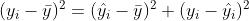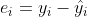Posted on:

24 Feb 2022

0

# How to "mathematically" prove this equation of sum of squares: SST = SSR + SSE

I am reading this 365 datascience article and I am stuck on the equation in this image:From what I understand, the above equation is the same as sayingbecauseAnd I can't find any way to prove that.

Can anyone shine a light on this?